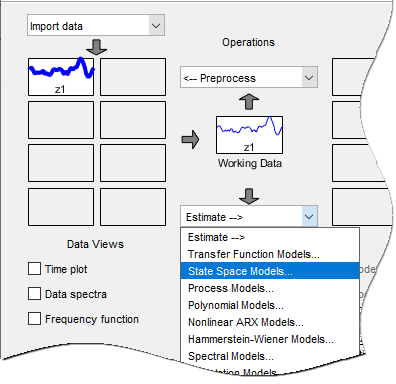## Estimate State-Space Models in System Identification App

1. Select Estimate > State Space Models.The State Space Models dialog box opens.### Tip

For more information on the options in the dialog box, click .

2. Specify a model name by clickingadjacent to Model name. The name of the model must be unique in the Model Board.

3. Select the Specify value option (if not already selected) and specify the model order in the edit field. Model order refers to the number of states in the state-space model.

### Tip

When you do not know the model order, search for and select an order. For more information, see Estimate Model With Selected Order in the App.

4. Select the Continuous-time or Discrete-time option to specify the type of model to estimate.

You cannot estimate a discrete-time model if the working data is continuous-time frequency-domain data.

5. Expand the Model Structure Configuration section to select the model structure, such as canonical form, whether to estimate the disturbance component (K matrix) and specification of feedthrough and input delays.6. Expand the Estimation Options section to select the estimation method and configure the cost function.

Select one of the following Estimation Method from the drop-down list and configure the options. For more information about these methods, see State-Space Model Estimation Methods.

7. Click to estimate the model. A new model gets added to the System Identification app.

### Assigning Estimation Weightings

You can specify how the estimation algorithm weights the fit at various frequencies. In the app, set Focus to one of the following options:

• `Prediction` — Uses the inverse of the noise model H to weigh the relative importance of how closely to fit the data in various frequency ranges. Corresponds to minimizing one-step-ahead prediction, which typically favors the fit over a short time interval. Optimized for output prediction applications.

• `Simulation` — Uses the input spectrum to weigh the relative importance of the fit in a specific frequency range. Does not use the noise model to weigh the relative importance of how closely to fit the data in various frequency ranges. Optimized for output simulation applications.

• `Stability` — Estimates the best stable model. For more information about model stability, see Unstable Models.

• `Filter` — Specify a custom filter to open the Estimation Focus dialog box, where you can enter a filter, as described in Simple Passband Filter or Defining a Custom Filter. This prefiltering applies only for estimating the dynamics from input to output. The disturbance model is determined from the estimation data.

##### SupportGet trial now